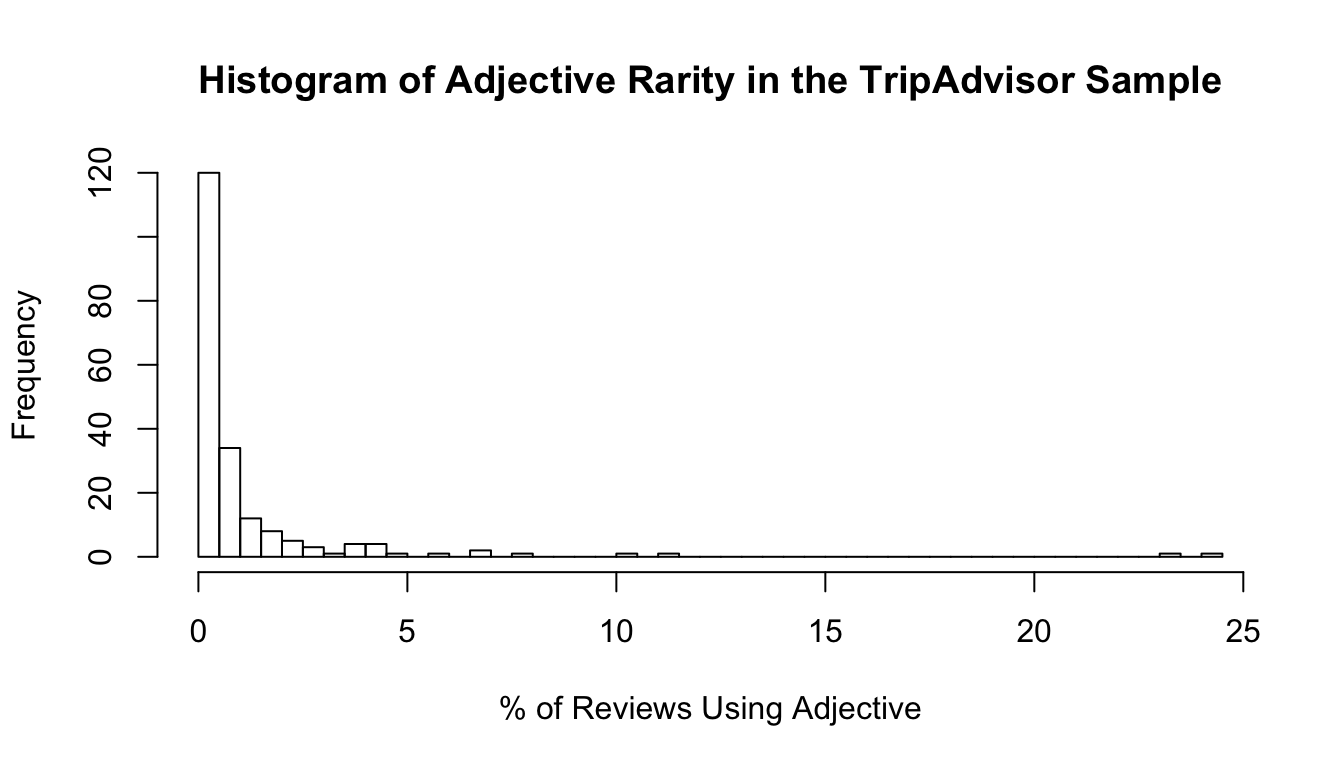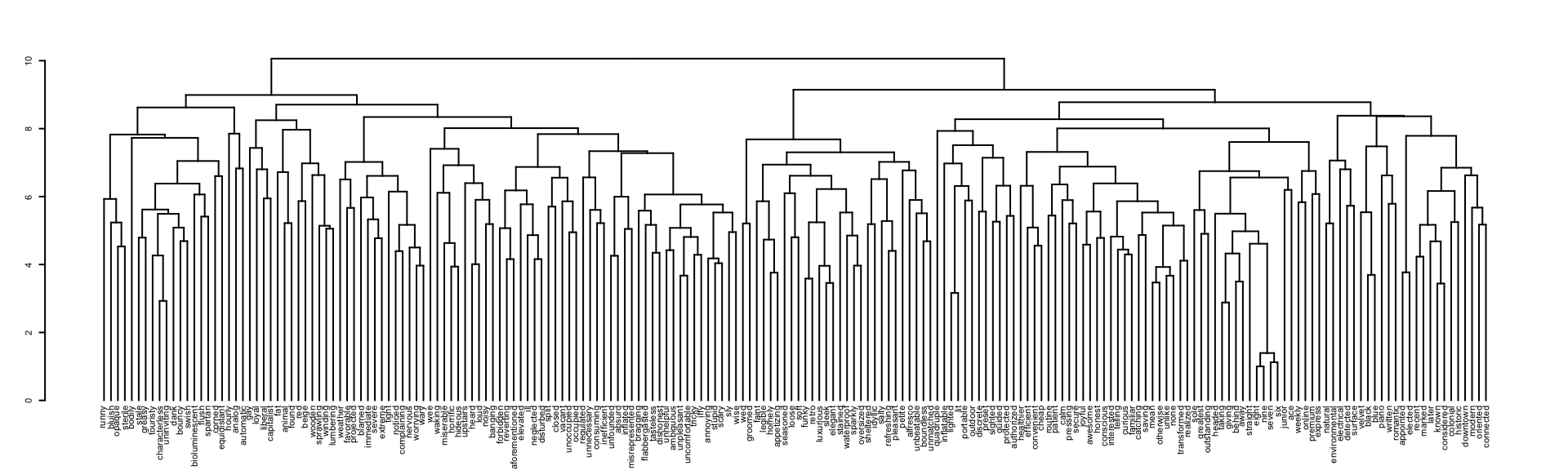Using the rare package

Xiaohan Yan

March 15, 2018

The rare package implements the rare feature selection procedure introduced in Yan, X. and Bien, J. (2018) Rare Feature Selection in High Dimensions, including fitting the model, performing K-fold cross validation, making predictions for new observations and visualizing aggregated groups of rare features in a colored dendrogram. In the following, we will use a real data example to demonstrate how to use the relevant functions.

The Problem

Rare features are hard to model because of their sparseness. For features measuring frequency of rare events, Yan and Bien (2018) proposes a regression framework for modeling the rare features. They use a tree as side information to relate $$p$$ features based on the similarity and aggregate them in a flexible manner with a tree-based parametrization strategy. The tree, denoted as $$\mathcal T$$ and with $$p$$ features’ coefficients $$\beta$$ on the leaves, merges two features at an earlier stage if they are more “similar”. The tree can be learned from a different data source or based on other prior knowledge. In every tree node, they assign a latent variable $$\gamma$$ and express $$\beta_j$$ on the $$j$$th leaf as a sum of all $$\gamma_u$$s that are ancestors of the $$j$$th leaf: $\beta_j = \sum_{u\in ancesotr(j)\cup \{j\}}\gamma_u.$ So sparsity in $$\gamma$$ induces fusion of $$\beta_j$$’s in a subtree. For notation conciseness, we represent the equality contraint between $$\beta$$ and $$\gamma$$ for a tree in a binary matrix $$A\in\{0, 1\}^{p\times |\mathcal T|}$$: $A_{jk} = 1_{\{u_k\in ancestor(j)\cup \{j\}\}}.$

Under a linear model $$y =\beta_0^*1_n+ X\beta^* + \epsilon$$ where $$y\in\mathbb R^n$$, $$X\in\mathbb R^{n\times p}$$ is the design matrix for $$p$$ features and $$\epsilon\sim N(0, \sigma^2I_n)$$, our proposed estimator $$\hat\beta$$ is the solution to the following optimization problem: $\min_{\beta\in\mathbb R^p, \gamma\in\mathbb R^{|\mathcal T|}}\left\{\frac{1}{2} \left\|y - X\beta - \beta_01_n \right\|_2^2 + \lambda \left(\alpha\left\|\gamma_{-root}\right\|_1 + (1-\alpha)\|\beta\|_1\right)\ \text{s.t. }\beta = A\gamma \right\}$ where $$\lambda$$ controls the overall regularization level while $$\alpha$$ determines the trade-off between fusion and sparsity in $$\hat\beta$$. In practice, both $$\lambda$$ and $$\alpha$$ are determined via cross validation. Refer to Yan and Bien (2018) for more details of the proposed framework.

A Data Example

To demonstrate the usage of rare, we use a review data set crawled from TripAdvisor.com (used in https://www.cs.virginia.edu/~hw5x/paper/rp166f-wang.pdf) as an example. The original data set contains more than 200 thousands reviews and ratings. . For the sake of the demonstration, we randomly subset 500 reviews and 200 adjectives appearing in them from the data set. In each review, user provides a rating on the scale ranging from 1 star to 5 stars. We model the rating with a Gaussian linear model: $$y_i = \beta_0^* + \sum_{j=1}^pX_{ij}\beta_j^* + \epsilon_i$$ where $$y_i$$ is the rating for the $$i$$th review, $$X_{ij}$$ counts the $$j$$th adjective in the $$i$$th review and $$\epsilon_i\sim N(0, \sigma^2)$$ i.i.d. for $$\sigma>0$$. We attach the sample data and a pre-trained hierarchical clustering tree to the package in data.rating, data.dtm and data.hc.

library(rare)
library(Matrix)
# Design matrix = document-term matrix
dim(data.dtm)
#>  500 200
# Ratings for the reviews in data.dtm
length(data.rating)
#>  500

The data set contains 200 adjectives in the sample and most of them are highly sparse. Below is a histogram of percentage of reviews using adjective.

hist(colMeans(sign(data.dtm)) * 100, breaks = 50, main = "Histogram of Adjective Rarity in the TripAdvisor Sample",
xlab = "% of Reviews Using Adjective")Our model relies on a hierarchical clustering tree as side information to guide feature aggregation. In the example, we generate the tree for adjectives in two steps: sentiment separation (negative and positive) and hierarchical clustering within each sentiment set. For sentiment separation, we use positive/negative emotion words from NRC Emotion Lexicon (Mohammad and Turney 2013) as train set to classify our adjectives to the two sentiments using 5NN. In the hierarchical clustering step, we apply hclust on 100-dimensional word embeddings for adjectives, which are pre-trained by GloVe (Pennington, Socher, and Manning 2014) on Gigaword5 and Wikipedia2014 corpora. The following dendrogram depicts the tree with 200 adjectives on the leaves.

par(cex=0.35)
plot(as.dendrogram(data.hc))Fit the Model

We split the sample data into training set and test set at the ratio of 4:1.

set.seed(100)
ts <- sample(1:length(data.rating), 400) # Train set indices

We let the program to determine $$\lambda$$ sequence and $$\alpha$$ sequence, after setting length of sequences to be nlam = 20 and nalpha = 10. We fit the model on the training set over the two-dimensional grid of regularization parameters $$(\lambda, \alpha)$$. The rarefit function implements the model fit alongside $$\alpha$$, i.e., at each $$\alpha$$ the model is fitted over the entire sequence of $$\lambda$$ values.

ourfit <- rarefit(y = data.rating[ts], X = data.dtm[ts, ], hc = data.hc, lam.min.ratio = 1e-6,
nlam = 20, nalpha = 10, rho = 0.01, eps1 = 1e-5, eps2 = 1e-5, maxite = 1e4)

rarefit provides various options for users to customize the fit. We introduce some commonly used options here and they can be specified in the rarefit function.

• A is a $$p$$-by-$$|\mathcal T|$$ binary matrix encoding ancestor-descendant relationships between leaves ($$\beta$$) and tree nodes ($$\gamma$$). If the tree $$\mathcal T$$ is not generated by hclust, user needs to provide A in a sparse matrix format (inherit from class sparseMatrix as in package Matrix). If $$\mathcal T$$ is generated by hclust, user can just provides the tree in hc.

• Q is a $$(p + |\mathcal T|)$$-by-$$p$$ matrix with columns forming an orthonormal basis for $$\begin{pmatrix}I_p: -A \end{pmatrix}$$. Computing Q can be time-consuming especially when $$p$$ is large. When fitting the model on the entire training set, user does not need to compute Q separately (i.e., leaving it NULL is fine). Later in cross validation, Q will be re-used every time the model is fitted on different folds of training set.

• intercept is a boolean value standing for whether intercept should be fitted. Default is TRUE. We recommend always including an intercept unless the data set has been centered.

• lambda can be provided, but is typically not and the program constructs a sequence. When automatically generated, the $$\lambda$$ sequence is determined by $$\lambda_{\max}$$ and lam.min.ratio. The former is the smallest $$\lambda$$ that sets all coefficients $$\beta$$ to zero. The latter is the smallest value for $$\lambda$$ as a fraction of $$\lambda_{\max}$$.

• alpha is another sequence of regularization parameters and can be provided. When automatically generated, the $$\alpha$$ sequence is a length-nalpha sequence of equally spaced values between 0 and 1. However, in practice user may find optimal $$\alpha$$ tends to be at a smaller region within [0, 1] interval. In that case, user may consider provide its own alpha sequence, e.g., alpha = c(1-exp(seq(0, log(1e-2), len = nalpha - 1)), 1)). is more granular towards 1.

• rho, eps1, eps2 and maxite are hyperparameters used in the ADMM algoirthm for solving our optimization problem. Refer to Algorithm 1 in Yan and Bien (2018) for details.

rarefit returns estimated coefficients $$\hat\beta_0$$, $$\hat\beta$$ and $$\hat\gamma$$ as length-nalpha lists: the $$j$$th entry in list corresponds to coefficients estimated at $$\alpha_j$$. In particular, $$\hat\beta_0[j]$$ is a length-nlambda vector with the $$i$$th entry being estimated intercept at $$(\lambda_i, \alpha_j)$$; $$\hat\beta[j]$$ is a $$p$$-by-nlambda matrix where the $$i$$th column being estimated $$\beta$$ at $$(\lambda_i, \alpha_j)$$; $$\hat\gamma[j]$$ is a $$|\mathcal T|$$-by-nlambda matrix where the $$i$$th column being estimated $$\gamma$$ at $$(\lambda_i, \alpha_j)$$. When $$\alpha= 0$$, our problem becomes the lasso on $$\beta$$ and rarefit returns NA value for $$\hat\gamma$$ (because we use glmnet to solve the lasso on $$\beta$$); for all other nonzero $$\alpha$$ values, $$\hat\gamma$$ are solved numerically.

Perform K-Fold Cross Validation

To choose optimal $$(\lambda, \alpha)$$ from the two-dimensional solution paths, we use K-fold cross validationt. The function rarefit.cv performs K-fold cross validation based on model fit from rarefit on the entire training set. rarefit.cv first randomly splits the training set into K folds that are roughly of the same size. At round $$k$$, rarefit.cv fits the model on all but the $$k$$th fold and predict on the $$k$$th fold, generating error metric $$errfun\left(y^{(k)}, \hat y^{(k)}(\lambda, \alpha)\right)$$. The optimal tuning parameter pair is the minimizer of an average of these metrics across K folds: $(\hat\lambda, \hat\alpha) = \arg\min_{\lambda, \alpha}\frac{1}{K} \sum_{k=1}^K errfun\left(y^{(k)}, \hat y^{(k)}(\lambda, \alpha)\right).$ An option that allows user to customize CV is errtype, a character string indicating the type of error function. Two error types are allowed: errtype = "mean-squared-error" or errtype = "mean-absolute-error". The default value for K is nfolds=5.

# Cross validation
ourfit.cv <- rarefit.cv(ourfit, y = data.rating[ts], X = data.dtm[ts, ],
rho = 0.01, eps1 = 1e-5, eps2 = 1e-5, maxite = 1e4)

Note that CV are done on the same sequences of $$\lambda$$ and $$\alpha$$ from the previous model fit in ourfit.

Make Predictions for New Observations

After choosing optimal $$(\lambda, \alpha)$$ using CV, we evalute our model’s performance on the hold-out test set (100 reviews and ratings from the sample). The function rarefit.predict is the one-click function for making new predictions, based on model fit object ourfit and CV object ourfit.cv (for choosing optimal $$(\lambda, \alpha)$$).

# Prediction on test set
pred <- rarefit.predict(ourfit, ourfit.cv, data.dtm[-ts, ])
pred.error <- mean((pred - data.rating[-ts])^2)
pred.error

The predictions are made at $$(\hat\beta_0({\hat\lambda}_{CV}, {\hat\alpha}_{CV}), \hat\beta({\hat\lambda}_{CV}, {\hat\alpha}_{CV}))$$, i.e., estimated regression coefficients $$(\hat\beta_0, \hat\beta)$$ from ourfit at the CV-chosen optimal $$(\hat\lambda_{CV}, \hat\alpha_{CV})$$.

Visualize Aggregated Groups in a Colored Tree

In addition to the prediction performance of the model, we may also be interested in seeing how the model aggregates rare adjectives into groups. We provide two functions to allow user view recovered groups at given $$(\hat\beta, \hat\gamma)$$: group.recover and group.plot.

The function group.recover determines aggregated groups of leaf indices (i.e., $$\beta$$ elements) based on sparsity in $$\gamma$$. In particular, we iterate over all non-zero $$\gamma$$ elements in postorder; at every non-zero $$\gamma$$, we make its descendant leaves a set after excluding all leaves that have appeared in previous groups. For example, suppose $$v_1$$ and $$v_2$$ are the only two children nodes of some node $$u$$ with $$\gamma_{v_1}\neq 0$$, $$\gamma_{v_2}=0$$, $$\gamma_{u} \neq 0$$ and $$\gamma_w = 0$$ for all $$w\in descendant(v_1)\cup descendant(v_2)$$. At node $$v_1$$, we recover $$\mathcal L(\mathcal T_{v_1})$$ (the leaf set of subtree rooted at $$v_1$$) as a group. Then we move to $$u$$ and recover $$\mathcal L(\mathcal T_{u})\backslash \mathcal L(\mathcal T_{v_1})$$ as a group. The postorder traversal across nodes with non-zero $$\gamma$$ ensures us recover the correct groups.

Since rarefit returns NA for $$\hat\gamma$$ when solving at $$\alpha = 0$$, group.recover (and the following group.plot) will only work for $$\alpha \neq 0$$ cases.

In the following, we find the groups aggregated at $$(\hat\beta_0({\hat\lambda}_{CV}, {\hat\alpha}_{CV}), \hat\beta({\hat\lambda}_{CV}, {\hat\alpha}_{CV}))$$.

# Find recovered groups at optimal beta and gamma
ibest.lambda <- ourfit.cv$ibest ibest.alpha <- ourfit.cv$ibest
beta.opt <- ourfit$beta[[ibest.alpha]][, ibest.lambda] gamma.opt <- ourfit$gamma[[ibest.alpha]][, ibest.lambda]
groups.opt <- group.recover(gamma.opt, ourfit$A) length(groups.opt) # total number of aggregated groups #>  32 In addition to a list of leaf indices representing aggregated groups, we can visualize the groups on a dendrogram. The function group.plot colors branches and leaves of an hclusttree based on corresponding $$\beta$$ values. In an hclust tree with $$\beta_i$$ on the $$i$$th leaf, the branch and leaf are colored in blue, red or gray according to $$\beta_i$$ being positive, negative or zero, respectively. The larger the magnitude of $$\beta_i$$ is, the darker the color will be. So branches and leaves from the same group will have the same color. In the following, we visualize the groups aggregated at $$(\hat\beta_0({\hat\lambda}_{CV}, {\hat\alpha}_{CV}), \hat\beta({\hat\lambda}_{CV}, {\hat\alpha}_{CV}))$$. # Visualize the groups at optimal beta and gamma par(cex=0.35) group.plot(beta.opt, gamma.opt, ourfit$A, data.hc)